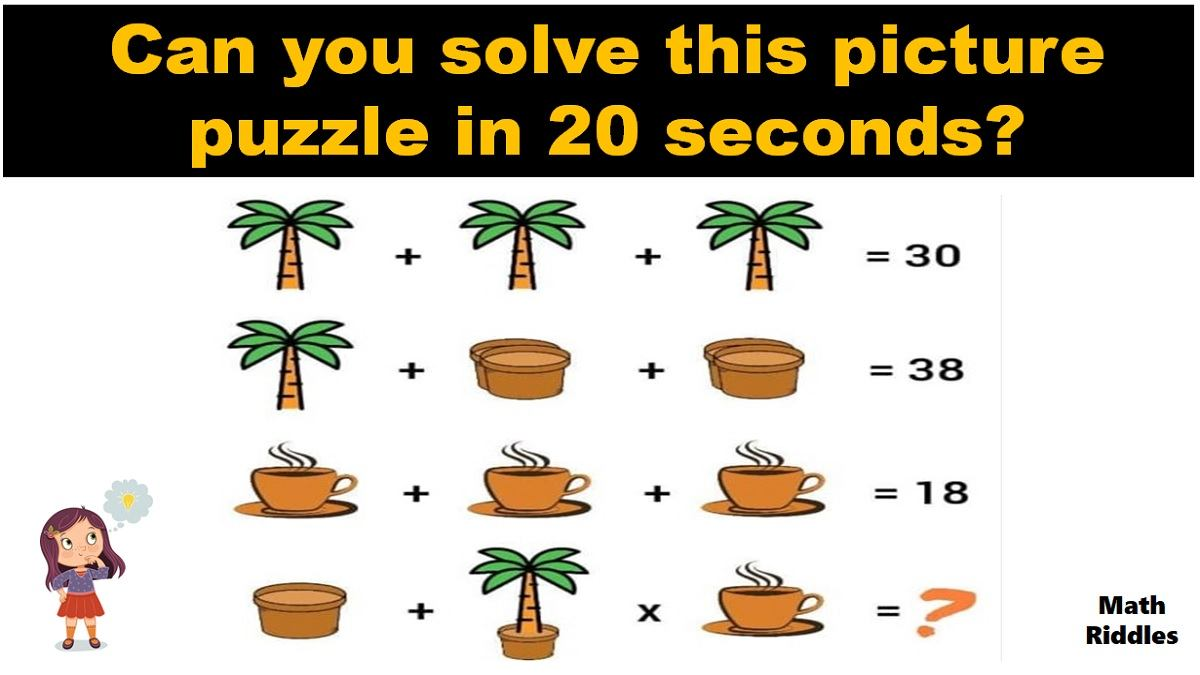# Math Riddles: Can You Solve This Picture Puzzle in 20 Seconds?

Math Picture Puzzles: Find out how brilliant you are. Only 1% of people with a high IQ can solve this tricky math picture puzzle in 20 seconds. Check out this math riddle for geniuses!Math Riddles: Can You Solve This Picture Puzzle in 20 Seconds?

Math Riddles are so challenging, but that makes them worthwhile to solve. Math riddles are logical problems that require strong analytical abilities, high IQ, knowledge of math concepts, and good calculation skills. Math riddles have also been known to make Mathematics enjoyable for kids, students, and even adults to solve. Riddles, puzzles, and brain teasers can develop strategic thinking, logical reasoning, and problem-solving skills.

Can you solve this math picture puzzle? You have 20 seconds!

## Math Picture Puzzle #1

Solution

Row 1: There are three coconut trees which equals to 30.

So, we get 3 coconut trees = 30, if we divide both sides by 3 then we get the result that 1 coconut tree is equal to 10. Now, we will use this value of 1 coconut tree to solve the next row of problems.

Row 2: Now, we have 1 coconut tree + two flowerpots + two flowerpots = 38. We know that 1 coconut tree equals 10, so we apply that and subtract from the total of 38. We get 28 which means that the value of these two flowerpots is 14 each and the value of one flowerpot is 7.

Row 3: There are three teacups which equals to 18.

So, we get 3 teacups = 18, if we divide both sides by 3 then we get the result that 1 teacup is equal to 6.

Therefore, so far, we have solved that the value of 1 coconut tree is 10, the value of 1 flowerpot is 7, and the value of 1 teacup is 6. Moving ahead, now we solve the next two rows to figure out the final answer to this picture puzzle.

Row 4: Now, we reach the final question of the puzzle where we need to find out what the total sum of the 1 flowerpot + 1 coconut tree with flowerpot + 1 teacup is. Hence, we apply the values of each item that we have ascertained from the previous rows of this puzzle to find out the final sum.

Now, this is where 99% of people fail to find the right answer. Notice, that the coconut tree is in a pot. Hence, we apply the values accordingly.

1 flowerpot + 1 coconut tree with flowerpot + 1 teacup =?

7 + (10 + 7) + 6 =?

7 + 17 + 6 = 109

## Tell us in comments: Did you solve these math puzzles correctly in 20 seconds each?

Check out more amazing tricky math puzzles!

Also Read: Math Riddle with Answer: Can You Solve These Math Puzzles in 20 Seconds?

Also Read: Math Riddle with Answer: Can You Solve These Tricky Math Puzzles?

Also Read: Math Riddles with Answers: Are you Genius? Find All Missing Numbers

Also Read: Math Riddles: Only High IQ Genius Can Solve These

Get the latest General Knowledge and Current Affairs from all over India and world for all competitive exams.
खेलें हर किस्म के रोमांच से भरपूर गेम्स सिर्फ़ जागरण प्ले पर